Download Presentation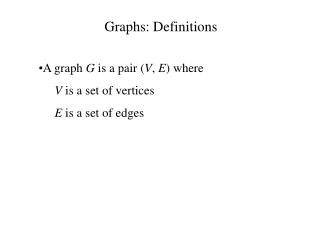Graphs: Definitions

# Graphs: Definitions - PowerPoint PPT Presentation

Graphs: Definitions A graph G is a pair ( V , E ) where V is a set of vertices E is a set of edges Graphs: Definitions A graph G is a pair ( V , E ) where V is a set of vertices V = {A, B, C, D, E, F}I am the owner, or an agent authorized to act on behalf of the owner, of the copyrighted work described.
Download Presentation## Graphs: Definitions

An Image/Link below is provided (as is) to download presentation

Download Policy: Content on the Website is provided to you AS IS for your information and personal use and may not be sold / licensed / shared on other websites without getting consent from its author.While downloading, if for some reason you are not able to download a presentation, the publisher may have deleted the file from their server.

- - - - - - - - - - - - - - - - - - - - - - - - - - E N D - - - - - - - - - - - - - - - - - - - - - - - - - -
Presentation Transcript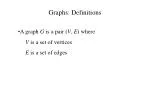Graphs: Definitions

• A graph G is a pair (V, E) where
• V is a set of vertices
• E is a set of edges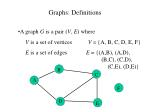Graphs: Definitions

• A graph G is a pair (V, E) where
• V is a set of vertices V = {A, B, C, D, E, F}
• E is a set of edges E = {(A,B), (A,D), (B,C), (C,D), (C,E), (D,E)}

B

C

A

F

D

E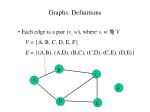Graphs: Definitions

• Each edge is a pair (v, w), where v, w V
• V = {A, B, C, D, E, F}
• E = {(A,B), (A,D), (B,C), (C,D), (C,E), (D,E)}

B

C

A

F

D

E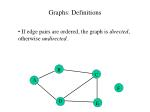Graphs: Definitions

• If edge pairs are ordered, the graph is directed, otherwise undirected.

B

C

A

F

D

E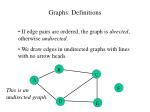Graphs: Definitions

• If edge pairs are ordered, the graph is directed, otherwise undirected.
• We draw edges in undirected graphs with lines with no arrow heads.

B

C

A

F

This is an

undirected graph.

D

E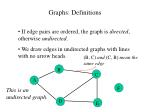Graphs: Definitions

• If edge pairs are ordered, the graph is directed, otherwise undirected.
• We draw edges in undirected graphs with lines with no arrow heads.

(B, C) and (C, B) mean the same edge

B

C

A

F

This is an

undirected graph.

D

E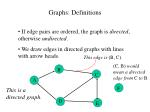Graphs: Definitions

• If edge pairs are ordered, the graph is directed, otherwise undirected.
• We draw edges in directed graphs with lines with arrow heads.

This edge is (B, C).

(C, B) wouldmean a directed edge from C to B

B

C

A

F

This is a

directed graph.

D

E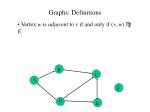Graphs: Definitions

• Vertex w is adjacent to v if and only if (v, w)  E.

B

C

A

F

D

E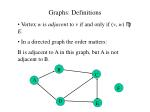Graphs: Definitions

• Vertex w is adjacent to v if and only if (v, w)  E.
• In a directed graph the order matters:
• B is adjacent to A in this graph, but A is not adjacent to B.

B

C

A

F

D

E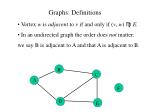Graphs: Definitions

• Vertex w is adjacent to v if and only if (v, w)  E.
• In an undirected graph the order does not matter:
• we say B is adjacent to A and that A is adjacent to B.

B

C

A

F

D

EGraphs: Definitions

• In some cases each edge has a weight (or cost) associated with it.

4

3

B

C

A

4.5

1.2

7.5

F

– 2.3

D

EGraphs: Definitions

• In some cases each edge has a weight (or cost) associated with it.
• The costs might be determined by a cost function, c: E  R, where Ris the real numbers (or integers).

4

3

B

C

A

4.5

1.2

7.5

F

– 2.3

D

EGraphs: Definitions

• In some cases each edge has a weight (or cost) associated with it.
• The costs might be determined by a cost function, c: E  R, where Ris the real numbers (or integers).

E.g., c(A, B) = 3,

c(D,E) = – 2.3, etc.

4

3

B

C

A

4.5

1.2

7.5

F

– 2.3

D

EGraphs: Definitions

• In some cases each edge has a weight (or cost) associated with it.
• When no edge exists between two vertices, we say the cost is infinite.

E.g., c(C,F) = 

4

3

B

C

A

4.5

1.2

7.5

F

– 2.3

D

EGraphs: Definitions

• Let G = (V, E) be a graph.
• A subgraph of G is a graph H = (V*, E*) such that V*  V and E*  E. (Since H is a graph, it must be the case that E*  V*  V*.)

B

C

A

F

D

EGraphs: Definitions

• Let G = (V, E) be a graph.
• A subgraph of G is a graph H = (V*, E*) such that V*  V and E*  E. (Since H is a graph, it must be the case that E*  V*  V*.)

E.g.,

V* = {A, C, D},

E* = {(C, D)}.

B

C

A

F

D

EGraphs: Definitions

• Let G = (V, E) be a graph.
• A subgraph of G is a graph H = (V*, E*) such that V*  V and E*  E. (Since H is a graph, it must be the case that E*  V*  V*.)

E.g.,

V* = {A, C, D},

E* = {(C, D)}.

C

A

DGraphs: Definitions

• Let G = (V, E) be a graph.
• A path in the graph is a sequence of vertices
• w , w , . . . , w such that (w , w )  E for1 i  N–1.

1

2

N

i

i+1

E.g.,

A, B, C, E

is a path in

this graph

B

C

A

F

D

EGraphs: Definitions

• Let w , w , . . . , w be a path.
• The length of the path is the number of edges, N–1, one less than the number of vertices in the path.

1

2

N

E.g., the length of

path A, B, C, E

is 3.

B

C

A

F

D

EGraphs: Definitions

• Let w , w , . . . , w be a path in a directed graph.
• Since each edge (w , w ) in the path is ordered,
• the arrows on the path are always directed along
• the path.

1

2

N

i

i+1

E.g., A, B, C, E

is a path in this directed graph,

but . . .

B

C

A

F

D

EGraphs: Definitions

• Let w , w , . . . , w be a path in a directed graph.
• Since each edge (w , w ) in the path is ordered,
• the arrows on the path are always directed along
• the path.

1

2

N

i

i+1

. . . but A, B, C, D

is not a path, since (C, D) is not an edge.

B

C

A

F

D

EGraphs: Definitions

A path is simple if all vertices in it are distinct, except that the first and last could be the same.

E.g., the

path A, B, C, E

is simple . . .

B

C

A

F

D

EGraphs: Definitions

A path is simple if all vertices in it are distinct, except that the first and last could be the same.

. . . and so is the

path A, B, C, E, D, A.

B

C

A

F

D

EGraphs: Definitions

A path is simple if all vertices in it are distinct, except that the first and last could be the same.

. . . but the

path A, B, C, E, D, C is not simple, since C is repeated.

B

C

A

F

D

EGraphs: Definitions

If G is an undirected graph, we say it is connected if there is a path from every vertex to every other vertex.

This undirected graph is not connected.

B

C

A

F

D

EGraphs: Definitions

If G is an undirected graph, we say it is connected if there is a path from every vertex to every other vertex.

This one is connected.

B

C

A

F

D

EGraphs: Definitions

If G is an directed graph, we say it is strongly connected if there is a path from every vertex to every other vertex.

This directed graph is strongly connected.

B

C

A

F

D

EGraphs: Definitions

If G is an directed graph, we say it is strongly connected if there is a path from every vertex to every other vertex.

This directed graph is not strongly connected; e.g., there’s no path

from D to A.

B

C

A

F

D

EGraphs: Definitions

If G is directed and not strongly connected, but the underlying graph (without direction to the edges) is connected, we say that G is weakly connected.

This directed graph is notstrongly connected, but it is

weakly connected, since . . .

B

C

A

F

D

EGraphs: Definitions

If G is directed and not strongly connected, but the underlying graph (without direction to the edges) is connected, we say that G is weakly connected.

. . . since the

underlying undirected graph is connected.

B

C

A

F

D

EGraphs: Definitions

• A cycle in a graph is a path of length at least 1 such that w = w .

1

N

The path

A, B, C, E, D, A

is a cycle.

B

C

A

F

D

EGraphs: Definitions

• A graph with no cycles is called acyclic.

This graph is

acyclic.

B

C

A

F

D

EGraphs: Definitions

• A graph with no cycles is called acyclic.

This directed graph is not acyclic, . . .

B

C

A

F

D

EGraphs: Definitions

• A graph with no cycles is called acyclic.

. . . but this one is.

A Directed Acyclic

Graph is often called

simply a DAG.

B

C

A

F

D

EGraphs: Definitions

• A complete graph is one that has an edge between every pair of vertices.

Incomplete:

B

C

A

D

EGraphs: Definitions

• A complete graph is one that has an edge between every pair of vertices.

Complete:

B

C

A

D

EGraphs: Definitions

• A complete graph is one that has an edge between every pair of vertices.
• Suppose G = (V, E) is complete. Can you express |E| as a function of |V|?

This graph has |V| = 5

vertices and |E| = 10 edges.

Complete:

B

C

A

D

EGraphs: Definitions

• A complete graph is one that has an edge between every pair of vertices.
• Suppose G = (V, E) is complete. Can you express |E| as a function of |V|?

Make a small table:

Complete:

|V|

|E|

1 0

AGraphs: Definitions

• A complete graph is one that has an edge between every pair of vertices.
• Suppose G = (V, E) is complete. Can you express |E| as a function of |V|?

Complete:

|V|

|E|

B

1 0

A

2 1Graphs: Definitions

• A complete graph is one that has an edge between every pair of vertices.
• Suppose G = (V, E) is complete. Can you express |E| as a function of |V|?

Complete:

|V|

|E|

B

C

1 0

A

2 1

3 3Graphs: Definitions

• A complete graph is one that has an edge between every pair of vertices.
• Suppose G = (V, E) is complete. Can you express |E| as a function of |V|?

Complete:

|V|

|E|

B

C

1 0

A

2 1

3 3

4 6

DGraphs: Definitions

• A complete graph is one that has an edge between every pair of vertices.
• Suppose G = (V, E) is complete. Can you express |E| as a function of |V|?

Complete:

|V|

|E|

B

C

1 0

A

2 1

3 3

4 6

5 10

D

EGraphs: Definitions

• A complete graph is one that has an edge between every pair of vertices.
• Suppose G = (V, E) is complete. Can you express |E| as a function of |V|?

|V|

|E|

B

C

1 0

A

2 1

3 3

F

4 6

5 10

D

E

6 ?Graphs: Definitions

• A complete graph is one that has an edge between every pair of vertices.
• Suppose G = (V, E) is complete. Can you express |E| as a function of |V|?

|V|

|E|

B

C

1 0

A

2 1

3 3

F

4 6

5 10

D

E

6 15Graphs: Definitions

• A complete graph is one that has an edge between every pair of vertices.
• Suppose G = (V, E) is complete. Can you express |E| as a function of |V|?

The pattern, for getting

from N–1 vertices to N,

is that we

N–1 new

edges . . .

|V|

|E|

B

C

1 0

+1

A

2 1

+2

3 3

F

+3

4 6

+4

5 10

D

+5

E

6 15Graphs: Definitions

• A complete graph is one that has an edge between every pair of vertices.
• Suppose G = (V, E) is complete. Can you express |E| as a function of |V|?

. . .so the

total number of edges,

when |V| = N

is . . .

|V|

|E|

B

C

1 0

+1

A

2 1

+2

3 3

F

+3

4 6

+4

5 10

D

+5

E

6 15Graphs: Definitions

• A complete graph is one that has an edge between every pair of vertices.
• Suppose G = (V, E) is complete. Can you express |E| as a function of |V|?

. . .so the

total number of edges,

when |V| = N

is

i = N(N – 1)/2

= |V|(|V| – 1)/2

|V|

|E|

B

C

1 0

+1

A

2 1

+2

N–1

3 3

F

+3

4 6

i = 0

+4

5 10

D

+5

E

6 15Graphs: Definitions

• A free tree is a connected, acyclic, undirected graph.

This is a free tree.

B

C

A

F

D

EGraphs: Definitions

• A free tree is a connected, acyclic, undirected graph.
• “Free” refers to the fact that there is no vertex designated as the “root.”

B

C

A

F

D

Eroot

Graphs: Definitions

• A free tree is a connected, acyclic, undirected graph.
• If some vertex is designated as the root, we have a rootedtree.

B

C

A

F

D

EGraphs: Definitions

• If an undirected graph is acyclic but possibly disconnected, it is a forest.

This is a forest. It contains three free trees.

H

K

G

B

C

A

I

F

J

L

D

EGraphs: Definitions

• If an undirected graph is acyclic but possibly disconnected, it is a forest.

This graph contains a cycle. Therefore it is neither a free tree nor a forest.

H

K

G

B

C

A

I

F

J

L

D

E